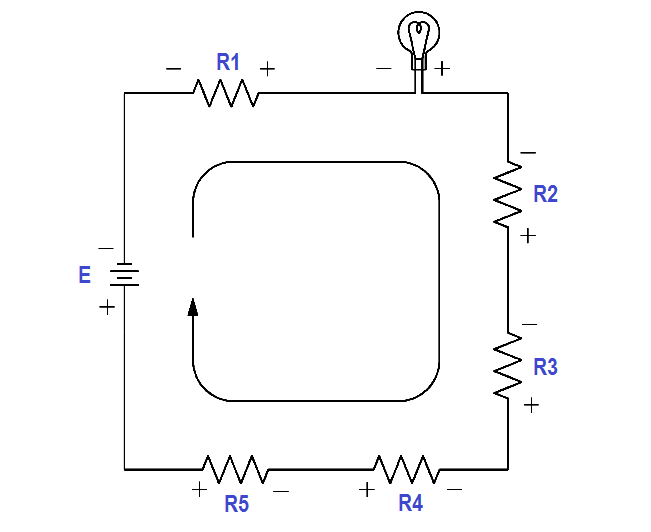# Voltage Polarity and Current Direction

Before understanding the laws associated with complex DC circuit analysis, the importance of voltage polarity and current direction must be understood. This article will introduce the polarities and current direction associated with DC circuits.

#### Conventional and Electron Flow

The direction of electron flow is from a point of negative potential to a point of positive potential. The direction of positive charges, or holes, is in the opposite direction of electron flow. This flow of positive charges is known as conventional flow.

All of the electrical effects of electron flow from negative to positive, or from a high potential to a lower potential, are the same as those that would be created by flow of positive charges in the opposite direction; therefore, it is important to realize that both conventions are in use, and they are essentially equivalent. In this manual, the electron flow convention is used.

#### Polarities

All voltages and currents have polarity as well as magnitude. In a series circuit, there is only one current, and its polarity is from the negative battery terminal through the rest of the circuit to the positive battery terminal. Voltage drops across loads also have polarities.

The easiest way to find these polarities is to use the direction of the electron current as a basis. Then, where the electron current enters the load, the voltage is negative (Figure 31). This holds true regardless of the number or type of loads in the circuit. The drop across the load is opposite to that of the source. The voltage drops oppose the source voltage and reduce it for the other loads. This is because each load uses energy, leaving less energy for other loads.Figure 31 Voltage Polarities

Be the first to get exclusive content straight to your email.
We promise not to spam you. You can unsubscribe at any time.

### 1 thought on “Voltage Polarity and Current Direction”

1.This would be more to the point in answering a simple query.
‘The direction of electron flow is from negative potential to positive potential.
The direction of conventional flow is positive potential to negative potential’.

Conventional and Electron Flow (present post)
The direction of electron flow is from a point of negative potential to a point of positive potential. The direction of positive charges, or holes, is in the opposite direction of electron flow. This flow of positive charges is known as conventional flow.